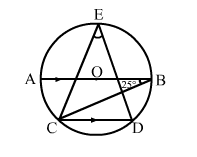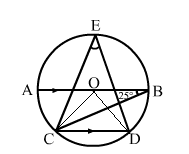# In the adjoining figure, O is the centre of a circle.

Question:

In the adjoining figure, O is the centre of a circle. Chord CD is parallel to diameter AB. If ABC = 25°, calculate ∠CED.Solution:BCD = ABC = 25° (Alternate angles)
Join CO and DO.
We know that the angle subtended by an arc of a circle at the centre is double the angle subtended by an arc at any point on the circumference.
Thus, BOD = 2BCD
BOD = 2 × 25° = 50°
Similarly, AOC = 2ABC
AOC = 2 × 25° = 50°
AB is a straight line passing through the centre.
i.e., AOC + COD + BOD = 180°
⇒ 50° + COD + 50° = 180°
⇒ COD = (180° – 100°) = 80°

$\Rightarrow \angle C E D=\frac{1}{2} \angle C O D$

$\Rightarrow \angle C E D=\left(\frac{1}{2} \times 80^{\circ}\right)=40^{\circ}$

∴ CED = 40°